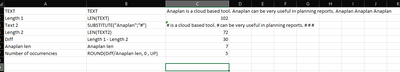# Count redundant word in a text line itemHi Team,

Is there a way to count a redundant words in a text line items? for example, I want to count the word "Anaplan" in a nested text line item that contains:

"Anaplan is a cloud based tool. Anaplan can be very useful in planning reports".

Is there a way to get a result "2" here.

Thank you,

Elaine

Tagged:

•@elaine.novel

There is no straightforward formula for this, but you can replicate it by various ways.

1) For example, using FIND()

This method means that you have to create as many line items as there are possible mentionings.

Each line item will search the next duplication. Until the return in one of them reaches =0

find x - is a line item

find 1 = FIND("Anaplan", <line item>,0)

find 2 = FIND("Anaplan", <line item>, 'find 1' +1)

find 3 = FIND("Anaplan", <line item>, 'find 2' +1)

and etc.

count duplicates = (if 'find 1' <>0 then 1 else 0) + (if 'find 2' <>0 then 1 else 0) + (if 'find 3' <>0 then 1 else 0)

(of course you can optimize the formulas, but now we are talking about a concept only)

The cons to this method is that many line items to be created (even repeated)

2) There is another solution. Using SUBSTITUTE() and LEN()

You can replace the key word by one symbol and then count length of the text before and after. The difference divided by len("Anaplan") (rounded up) - will return the occurrencies number